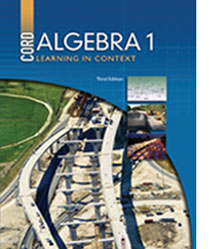# Algebra - 3rd Edition

Some links are repeated for use with more than one lesson.

### This site applies to the entire chapter.

This site defines a quadratic function and a quadratic equation and shows examples of each.

NEW An illustrated tutorial on graphing quadratic equations, including how to interpret and use the general quadratic form.

http://www.explorelearning.com/index.cfm?method=cResource.dspResourcesForCourse&CourseID=245
This site contains gizmos that show the quadratic function and inequalities.

This site shows how to find the sign of a quadratic function.

### 11.3 Solving a Quadratic Equation by Factoring

This site contains notes on solving quadratic equations by factoring and includes examples and practice problems.

### 11.4 Completing the Square

This page contains a video tutorial with notes covering completing the square.

http://www.mathsisfun.com/algebra/completing-square.html
This site contains description on how to complete the square with several examples on different cases of problems.